Courses

# Structural Requirements, Code Stipulations and Governing Equations Civil Engineering (CE) Notes | EduRev

## Civil Engineering (CE) : Structural Requirements, Code Stipulations and Governing Equations Civil Engineering (CE) Notes | EduRev

The document Structural Requirements, Code Stipulations and Governing Equations Civil Engineering (CE) Notes | EduRev is a part of the Civil Engineering (CE) Course RCC & Prestressed Concrete.
All you need of Civil Engineering (CE) at this link: Civil Engineering (CE)

Instructional Objectives: At the end of this lesson, the student should be able to:

• state the need to design the tension structures using reinforced concrete though concrete is very weak in tension,
• justify the use of working stress method for designing reinforced concrete tension members,
• name the two essential requirements for the design of reinforced concrete tension structures,
• explain why some amount of cracking may be allowed in such structures,
• explain why porous aggregates are not allowed in the reinforced concrete tension structures,
• state the basis of design of reinforced concrete tension members,
• state the different cases of reinforced concrete tension members, and
• establish the governing equations for all the cases of tension members to determine the stresses of concrete and steel following the working stress method of design.

14.36.1  Introduction

Reinforced concrete is used in the design of several practical members of tension structures though it is not a potential material due to very inadequate tensile strength of concrete compared to its compression strength. In such cases, reinforced concrete develops tensile stresses either due to direct tension force or combined with bending. Tie members of trusses and arches, walls of rectangular tanks and bunkers, suspended roofs, cylindrical pipes, walls of liquid retaining structures are some of the examples where tension stresses also develop. Traditional elastic approach (working stress method) and the limit state method can be applied for the design of such structures. Based on elastic theory, the working stress method is simpler in concept and applications, while the limit state method, based on cracking behaviour of concrete, is not fully developed yet.

Some of the structures or members mentioned above may not be in direct contact with any liquid. On the other hand, some may be in direct contact with liquid. Though control of cracking is important for all types of structures, it is an essential requirement for liquid retaining structures. Impermeability of structure is not only for preventing leakage but also for improving durability, resistance to leaching, chemical action, erosion, frost damage and protecting the reinforcement from corrosion.

IS 456 gives due considerations for compression either direct or associated with bending and shear when limit state method is employed. However, it is almost silent on tension members. IS 3370, dealing with liquid retaining structures, is still adopting working stress method. Thus, the applications of limit state method for the design of tension members are still in the development stage. Accordingly, the working stress method shall be employed in this lesson to explain the design of such members.

14.36.2  Resistance to Cracking and Strength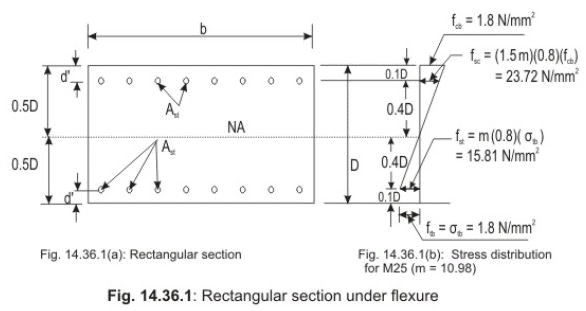Figures 14.36.1a and b show one rectangular section under flexural tension and the distribution of stresses, respectively. The symmetric section of depth D is having the neutral axis at a depth of 0.5 D. Assuming that the actual tension stress of M 25 concrete (say) in bending ftb reaches the permissible value of σtb = 1.8 N/mm2 for resistance to cracking (see Table 14.1), the tensile stress of steel fst at the level of steel shall be modular ratio times 0.8 σtb or 15.81 N/mm2 {(10.98) (0.8) (1.8) N/mm2, where modular ratio of M 25 concrete m = 93.33 / 8.5 = 10.98 and d′ = 0.1 D}. The value of fst is very low when compared with the values of the permissible tension stress of steel (115 N/mm2 for Fe 250 and 150 N/mm2 for Fe 415 as given in Table 14.2. Therefore, some amount of cracking may be permitted when the structure is not in contact with any liquid at all. Moreover, the remote face of the liquid retaining structures, which is not in contact with the liquid, also is a possible place of allowing minor cracks. However, it is also important to note that sufficient depth is available to prevent the corrosion of the reinforcement.

Allowing minor cracks in such cases means increasing the values of permissible stresses of concrete and steel reinforcement specifying the particular cases. IS 3370 Part II recommends the permissible stresses of concrete and reinforcement for different types of situations, which are given in Tables 14.1 and 14.2.

Hence, the design of tension members shall provide adequate control of cracking in addition to normal requirements of strength. There are many other issues involved in the design of tension structures. IS 3370 Parts I to IV deal with such issues in detail. The relevant important aspects are briefly mentioned in the following section.

14.36.3  IS Code Stipulations

(a)  Porous aggregates – (cl.2.1.a of IS 3370, Part I)  Use of porous aggregates, such as burnt clay and broken bricks or tiles is not allowed for parts of structure either in contact with the liquids on any face or enclosing the space above the liquid.

(b) Concrete mix - (cl.3.1 a of IS 3370, Part I)  Concrete mix weaker than M 20 shall not be used except in thick sections (thickness > 450 mm) and parts of structure neither in contact with the liquid on any face nor enclosing the space above the liquid.

(c) Basis of design - (cl.3.2 of IS 3370, Part II)

(i) The parts of the structure not in contact with the liquid shall be designed in accordance with the requirements of IS 456.

(ii) Design of members other than those in (i) above shall be based on consideration of adequate resistance to cracking as well as adequate strength.

(iii) Calculation of stresses shall be based on the following assumptions in addition to the general assumptions given in IS 456.

1. The whole section of concrete including the cover together with the reinforcement shall be taken into account in calculating both flexure and direct tension (or combination of both) relating to resistance to cracking.

2. Total shear stress = Q/(b jd), shall not exceed the permissible value given in Table 14.1, where Q, b and jd are the total shear, breadth and lever arm, respectively.

3. Concrete has no tensile strength in strength calculations.

(d) Permissible stresses in concrete - (cl.3.3 of IS 3370 Part II)

(i) For resistance to cracking

able 14.1 furnishes the permissible stresses in tension σtd and σtb (direct and due to bending) and shear τsh for calculations relating to the resistance of members to cracking. The permissible tensile stresses due to bending apply to the face of the member in contact with the liquid. In members less than 225 mm thick and in contact with the liquid on one side, these permissible stresses in bending apply also to the face remote from the liquid.

Table 14.1: Permissible stresses in concrete in calculations relating to resistance to cracking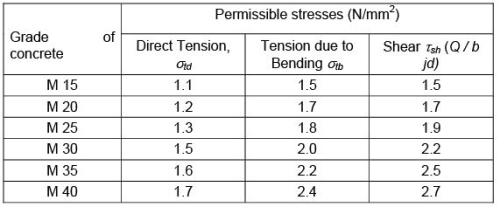(ii) For strength calculations

In strength calculations, the permissible concrete stresses shall be in accordance with IS 456 (Table 13.1 of Lesson 34). Where the calculated shear stress in concrete alone exceeds the permissible value, reinforcement acting in conjunction with diagonal compression in the concrete shall be provided to take the whole of the shear.

(e) Permissible stresses in steel - (cl.3.4 of IS 3370, Part II)

(i) For resistance to cracking

When steel and concrete are assumed to act together for checking the tensile stress in concrete for avoidance of cracks, the tensile stress in the steel will be limited by the requirement that the permissible tensile stress in concrete is not exceeded, so that the tensile stress in steel shall be equal to the product of modular ratio of steel and concrete and the corresponding allowable tensile stress in concrete.

(ii) For strength calculations

For strength calculations the permissible stresses in steel reinforcement are given in Table 14.2.

Table 14.2: Permissible stresses in steel reinforcement for strength calculations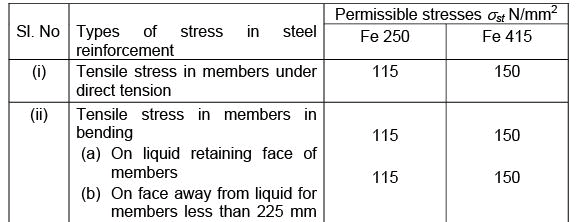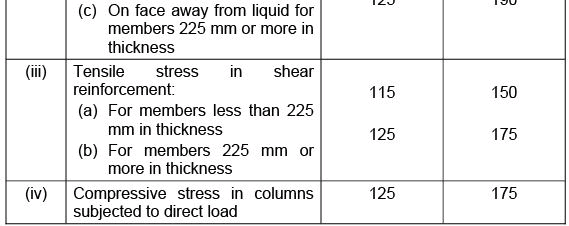Note: Stress limitations for liquid retaining faces shall also apply to the following:

a) Other faces within 225 mm of the liquid retaining face.

b) Outside or external faces of structures away from the liquid but placed in water logged soils up to the level of the highest subsoil water.

14.36.4  Types of Tension Structures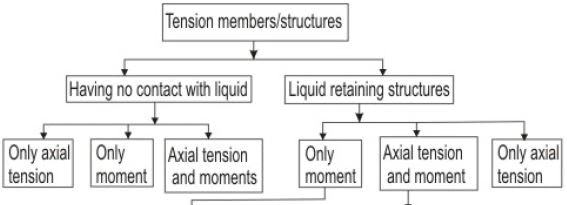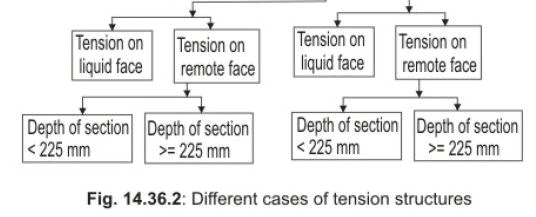Figure 14.36.2 summarises the two types of tension structures made of reinforced concrete indicating the different cases. The two types are (i) structures having no contact with the liquid and (ii) structures in direct contact with the liquid or liquid retaining structures. The different cases are first due to the three sources of tension stresses: (i) due to direct tension, (ii) pure flexure and (iii) direct tension associated with the flexure. Secondly, for the liquid retaining structures if the tension is on the (i) liquid face or (ii) on the remote face. Further two cases are there for the structures having tension on the remote face depending on, if the depth of section is (i) < 225 mm or (ii) ≥ 225 mm.

It is worth mentioning that understanding of the behaviour of tension structures and developing the governing equations of different cases are possible by taking up three main types: (i) when subjected to axial tension only, (ii) when subjected to bending moment only and (iii) when subjected to combined axial tension and bending moment. Accordingly, we take up these three main types in the following sections.

14.36.5  Members Subjected to Axial Tension only

Based on the discussion made so far, the criteria of design of such tension members are:

(i) Reinforcement alone shall take the full tension force.

(ii) The tension stress in steel shall not exceed the recommended permissible stress values given in Table 22 of IS 456 and Table 13.2 of Lesson 34.

(iii) The tensile stress of concrete shall be determined transforming the steel into equivalent concrete.

(iv) The tensile stress in the transformed section shall not exceed the stipulated permissible values given in cl. B-2.1.1 of IS 456 (Table 13.1 of Lesson 34). This will regulate the cracking of concrete.

Therefore, we can determine the actual tensile stress of steel fst and concrete ftd from the following equations:

fst = Ft / Ast        (14.1)

and  ftd = Ft /(A+ m Ast)  (14.2)
where

F= total tension force on the member minus pretension in steel, if any, before concreting,

Ast = cross-sectional area of reinforcing steel in tension,

Ac = cross-sectional area of concrete excluding any finishing material and reinforcing steel, and

m =  modular ratio

14.36.6  Members Subjected to Pure Flexure

For this, we will consider two cases:
(i) when the section is uncracked and
(ii) when the section is cracked. The first case is applicable when either the tension is on the liquid face or when the reinforcement is away from the liquid by a distance less than 225 mm. The second case is applicable when there is no direct contact of the structure with the liquid. The two cases are explained below.

(A) Uncracked Section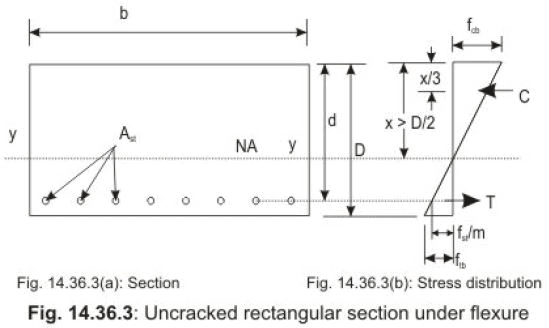Figures 14.36.3a and b show the uncracked section and the stress distribution of such section subjected to bending moment alone. The maximum bending stresses in compression or tension are given by the expression.

fcb = M x / Iyy        (14.3)

and  ftb = M  (D-x) / Iyy       (14.4)

where  M  =  Applied moment on the member

x and (D - x) = maximum distances of the compression and tension fibres from the neutral axis, x is also the depth of the neutral axis.

Iyy  = moment of inertia of the uncracked section about the neutral axis of the section transforming area of steel into equivalent concrete.

The depth of the neutral axis x is obtained by taking moment of the transformed section about the top face, which gives: bD2/2 + (m-1) pt b Dd/100 = {bD + (m-1) pt bD / 100}x

or  x = {b D2/2 + (m –1) pt b Dd/100} / {bD + (m-1) pt bD / 100} (14.5)

The moment of inertia of the transformed section about the neutral axis is obtained from:

Iyy = b x3 / 3 + b (D – x)3 / 3 + (m –1) pt bD (D-x)2/100  (14.6)

The values of fcb and ftb are then obtained from Eqs. 14.3 and 14.4, respectively.

The moment of resistance of the section is determined from Eq.14.4 as ftb is the governing stress of the section. This gives

M = ftb Iyy / (D – x)       (14.7)

(B) Cracked Section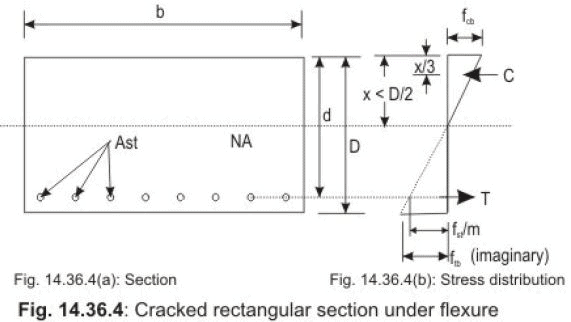Figures 14.36.4a and b show the section and stress distribution of the cracked section when subjected to moment alone. This particular case has been discussed in secs. 13.34.8 and 13.34.9 when the section is balanced and underreinforced, respectively. Therefore, the equations are not derived here and only the final equations are given for the balanced and under-reinforced sections separately. The only difference is the notation of compressive stress of concrete in bending compression, which is fcbc in secs. 13.34.8 and 9, while the same is denoted by fcb  here.

(i) Equations for the under-reinforced sections

The depth of the neutral axis is determined from x = kd, where

k = - (pt m / 100) + {(ptm / 100)2 + (pt m / 50)}1/2  (13.16)

The compressive and tensile forces C and T are obtained from:

C = (1/2) fcb b kd  (13.20)

T = fst Ast (13.6)

The moment of resistance with respect to steel is obtained from:

M = Ast fst d(1 – k/3)        (13.24)

M = (pt /100)fst (1 – k/3) bd2  (13.19)

(ii) Equations for the balanced section are as follows:

The depth of the balanced neutral axis is determined from: xb = kb d, where

kb = 93.33 / (σ st + 93.3 3 )       (13.3)

The expressions of compressive and tensile forces C and T are:

C = (1/2) (σcb) b kb d       (13.5)

T = Ast σ st         (13.6)

The expressions of the balanced moment of resistance with respect to concrete and steel are:

Mb = (1/2) σ cb kb j(b d 2)       (13.7)

Mb = (pt, bal / 100) σ st jb (b d2)       (13.8)

14.36.7  Members Subjected to Combined Axial Tension and Moment.

Here also, we will consider two cases: (i) when the section is uncracked and (ii) when the section is cracked.

(A) Uncracked Section

If tension is there on the liquid face, the tensile stress actually developed, i.e., ftd and ftb in direct tension and bending tension, respectively should satisfy the following condition:

(ftdtd) + (ftb / σ tb)≤ 1.0        (14.8)

where σtd and σtb are the permissible stresses of concrete in direct tension and bending tension, respectively and are to be taken from Table 14.1, and ftd and ftb are the actual stresses of concrete in direct tension and bending tension, respectively and are determined from Eqs.14.2 and 14.4, respectively.

(B) Cracked Section

This case is classified under two groups: (i) when the eccentricity e of the tension force Ft is small; i.e., tensile force is large and (ii) when the eccentricity e of the tension force is large, i.e., tension force is small. We are explaining them separately.

(i) When the eccentricity is small, i.e., Ft is large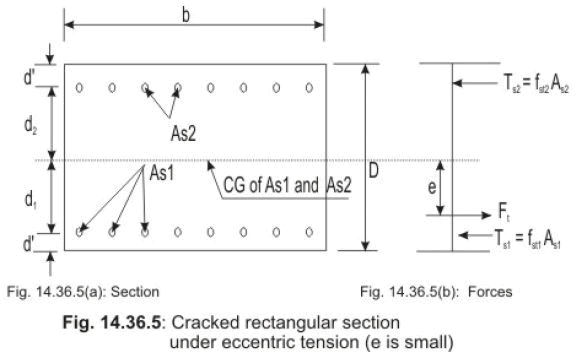Figures 14.36.5a and b show the cracked section subjected to tension force at a small eccentricity e within the depth of the section. As the eccentricity is small, measured from the center of gravity of the two areas of steel, As1 and As2, the whole section is in tension and hence the entire concrete is ineffective.

We have two equations of equilibrium: (i) equilibrium of forces and (ii) equilibrium of moments. They are expressed as follows:

F= Ts1 + Ts2 = fst1 As1 + fst2 As2      (14.9)

Moment of the forces about the bottom steel gives:  Ft (d1 – e) – Ts2 (d1 + d2) = 0

or Ft (d– e) – fst2 As2 (d1 + d2) = 0  (14.10)

Substituting the expression of Ft from Eq.14.9 into Eq.14.10, fst2 can be computed. The value of fst1 can then be computed from Eq.14.9.

(ii) When the eccentricity is large, i.e., Ft is small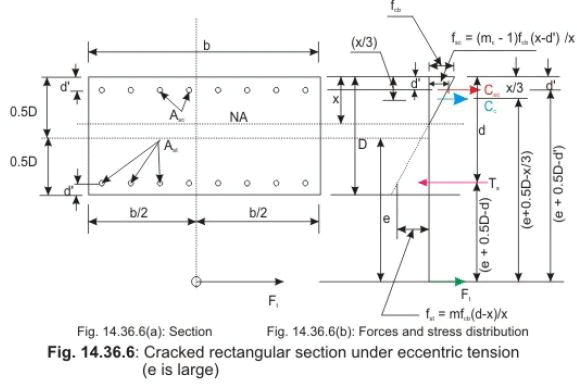Figure 14.36.6a and b show the section and stress distribution of cracked section subjected to tension force Ft having large value of the eccentricity e measured from the mid-depth of the section. The line of action of Ft is outside the section. The areas of compression and tension steel are denoted by Asc and Ast, respectively. Due to large eccentricity, the direct and bending stresses are equally dominant. The forces of compression and tension steel are represented by Csc and Ts, respectively, while the force of compressive concrete is represented by Cc. The distance of the neutral axis is x.

The expressions of these three forces are: Csc = fsc Asc  (14.11)
Ts = fst Ast  (14.12)
C= 0.5 fcb bx  (14.13)
The expressions of fsc and fst, obtained from the stress distribution of Fig. 14.36.6 b, are:

fsc = (mc –1) fcb (x - d′ )/x  (14.14)
fst = m fcb (d – x) / x  (14.15)

Using the expression of fsc and fst from Eqs. 14.14 and 14.15 in Eqs. 14.11 and 14.12, we have:

Csc = (mc – 1) Asc fcb (x - d′ ) / x  (14.16)
Ts = m Ast  fcb (d – x) / x  (14.17)

The equation of the equilibrium of forces is:

F– Ts + Cc + Cse = 0        (14.18)

Taking moment of all forces about the line of action of Ft, the equation of equilibrium of moment is:

T(e + 0.5D - d) – Cc (e + 0.5D – x/3) – Csc (e + 0.5D - d′ ) = 0  (14.19)

Substituting the expressions of Cc, Csc and Ts from Eqs. 14.13, 14.16 and 14.17, respectively into Eqs. 14.18 and 14.19, we have:

Ft = fcb {m Ast (d – x) / x – 0.5 bx – (mc – 1)Asc (x -d′ ) / x}   (14.20)

m Ast (d –x) (e + 0.5D –d) / x = 0.5 bx (e + 0.5D – x/3) + (mc – 1) Asc (x-d′) (e +    0.5D - d′) / x (14.21)

Equations 14.20 and 14.21 have seven unknowns b, d, D, x, fcb, Ast and Asc of which b, d  and D are assumed or taken from the preliminary dimensions. So, we have four unknowns x, fcb, Ast and Asc to be determined from two equations (Eqs. 14.20 and 14.21). Therefore, trial and error method has to be used.

As a particular case, when Asc = 0, Eqs. 14.20 and 14.21 are reduced to:

Ft = fcb {m Ast (d –x) / x – 0.5 bx}      (14.22)

and    m Ast (d – x) (e + 0.5 D – d) / x = 0.5 bx (e + 0.5 D – x/3)   (14.23)

Here also the three unknowns x, fcb and Ast are determined from Eqs. 14.22 and 14.23 by trial and error.

However, for such problems of singly-reinforced section, the initial value of Ast can be determined as explained below.

We use the equation of equilibrium of forces i.e., Eq. 14.24 as such and write the equation of equilibrium of moment by taking moment about Ts, which gives

Cc (jd) – Ft (e + 0.5D – d) = 0      (14.24)
or C= Ft (e + 0.5 D – d)/jd  (14.25)

Using the expression of Cc from Eq. 14.25 in Eq. 14.18, when Asc = 0, we get:

Ft = Ts - C     =  Ts – Ft (e + 0.5 D – d)/ jd

or Ts  =F{1 + (e + 0.5 D –d)/ jd}      (14.26)

Assuming Ts = Ast σ st in Eq. 14.26, we have:

Ast = (Ft / σst){1+ (e + 0.5 D – d)/ jd}     (14.27)

Equation 14.27 is used to get the starting value of Ast assuming j as 0.87 (say). This value of Ast now can be used in Eq. 14.23 to get the value of x. Using that value of x in Eq. 14.22, the value of fcb can be determine. If fcbcb, either the value of Ast shall be increased keeping D as the same or the value of D may be increased keeping Ast as the same. The third option is to increase both Ast and D, as appropriate.

Numerical problems are taken up in Lesson 37 for tension structures having no contact with liquid and for liquid retaining structures covering all the cases explained in Fig. 14.36.2.

Summary of this Lesson

This lesson presents the analysis and design of tension structures following the working stress method as stipulated in IS 456 and IS 3370. The two requirements i.e., the resistance to cracking and strength must be considered for all types of tension structures whether they are in direct contact with the liquid or not. The respective permissible stresses of concrete in direct tension, bending tension, bending compression and shear are taken from the IS Codes for both cases: when no cracking is allowed and when some cracking may be allowed. The different cases of tension structures are classified depending on if they are in direct contact with the liquid or not, if the tension is on the liquid face or not and on the depth of the section. Understanding of the philosophy and concept of design and the different equations established for different cases will help to apply them in numerical problems. The numerical problems are taken up in Lesson 37.

Offer running on EduRev: Apply code STAYHOME200 to get INR 200 off on our premium plan EduRev Infinity!

## RCC & Prestressed Concrete

14 videos|24 docs|12 tests

,

,

,

,

,

,

,

,

,

,

,

,

,

,

,

,

,

,

,

,

,

,

,

,

;